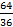Home Technical Mathematics Languages Science Social Science Art Literature Arabic Islamic Studies C.R.K HistoryMusicVisual Art Clothing/Textile Home Management Shorthand
 General Mathematics Paper 2, Nov/Dec. 2009
 Questions: 1 2 3 4 5 6 7 8 9 10 11 12 13 Main
Weakness/Remedies
Strength
Question 13

(a)        Without using tables or calculator, evaluate     0.0028 x 90

(b)  The pilot of an aircraft 2,000 metres above the sea level observes at an instant that the angles of depressions of two boats which are in direct straight line are 58° and 72°.  Find correct to the nearest metre, the distance between the two boats.

Part (a) of the question involved finding the square root of decimal numbers.
Candidates were expected to convert these numbers to whole numbers, simplifying them and take the square root.  However, majority of the candidates were reportedly unable to do this, but rather resorted to using calculators which was not required of them.  Multiplying both the numerator and the denominator by 1000 gave   14 x 32
28 x 9
Simplifying this resulted inwhich gave 8/6 =11/3

_____________________________________________________________________________________________________
Observation

In part (b), many candidates could not draw the diagram correctly.  Many others could not differentiate between angles of elevation and depression.  They were expected to interpret the question diagrammatically as:In the diagram, M and N represents the two boats.  /XM/ = 2000 tan 18° = 649.84cm, /XN/ = 2000 tan 32° = 1249.74.  /MN/ = 1249.74 – 649.84 = 600 m to the nearest m.  In general, it was reported that majority of the candidates attempted this question and their performance were quite commendable.

The report stated that questions 14 and 15 were not attempted by the students as was demanded by the rubrics of the question.